#### Bounded speed

There has been no consideration so far of the speed at which the robot must move to avoid obstacles. It is obviously impractical in many applications if the solution requires the robot to move arbitrarily fast. One step toward making a realistic model is to enforce a bound on the speed of the robot. (More steps towards realism are taken in Chapter 13.) For simplicity, suppose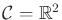, which corresponds to a translating rigid robot,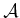, that moves in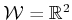. A configuration,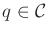, is represented as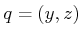(sincealready refers to the whole state vector). The robot velocity is expressed as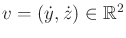, in which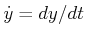and. The robot speed is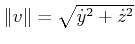. A speed bound,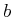, is a positive constant,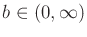, for which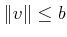.

In terms of Figure 7.1, this means that the slope of a solution path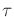is bounded. Suppose that the domain ofis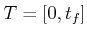instead of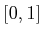. This yields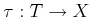and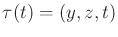. Using this representation,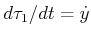and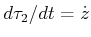, in which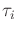denotes theth component of(because it is a vector-valued function). Thus, it can seen thatconstrains the slope of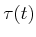in. To visualize this, imagine that only motion in the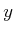direction occurs, and suppose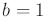. Ifholds the robot fixed, then the speed is zero, which satisfies any bound. If the robot moves at speed, then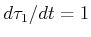and, which satisfies the speed bound. In Figure 7.1 this generates a path that has slopein the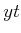plane and is horizontal in the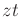plane. If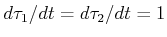, then the bound is exceeded because the speed is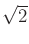. In general, the velocity vector at any state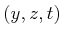points into a cone that starts at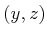and is aligned in the positive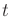direction; this is depicted in Figure 7.3. At time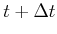, the state must stay within the cone, which means that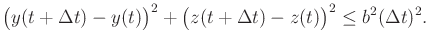(7.4)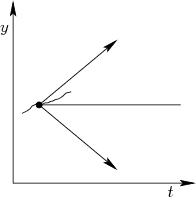This constraint makes it considerably more difficult to adapt the algorithms of Chapters 5 and 6. Even for piecewise-linear motions of the obstacles, the problem has been established to be PSPACE-hard [818,819,928]. A complete algorithm is presented in  that is similar to the shortest-path roadmap algorithm of Section 6.2.4. The sampling-based roadmap of Section 5.6 is perhaps one of the easiest of the sampling-based algorithms to adapt for this problem. The neighbors of point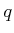, which are determined for attempted connections, must lie within the cone that represents the speed bound. If this constraint is enforced, a resolution complete or probabilistically complete planning algorithm results.

Steven M LaValle 2020-08-14